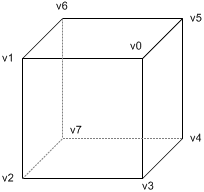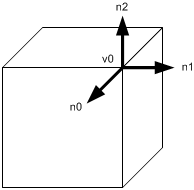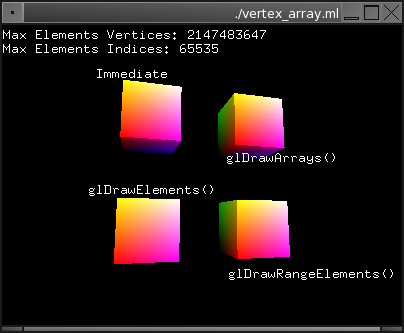The original version of this document for the C language is available there →

# OpenGL Vertex Array in OCaml

Related Topics: Vertex Buffer Object, Display List
Source Code: vertex_array.ml

Instead you specify individual vertex data in immediate mode (between glBegin() and glEnd() pairs), you can store vertex data in a set of arrays including vertex coordinates, normals, texture coordinates and color information. And you can draw geometric primitives by dereferencing the array elements with array indices.Take a look the following code to draw a cube with immediate mode.

Each face needs 4 times of glVertex3() calls to make a quad, for example, the quad at front is v0-v1-v2-v3. A cube has 6 faces, so the total number of glVertex3() calls is 24. If you also specify normals and colors to the corresponding vertices, the number of function calls increases to 3 times more; 24 of glColor3() and 24 of glNormal3().

The other thing that you should notice is the vertex "v0" is shared with 3 adjacent polygons; front, right and up face. In immediate mode, you have to provide the shared vertex 3 times, once for each face as shown in the code.

```glBegin GL_QUADS;     (* draw a cube with 6 quads *)
glVertex3v v0;    (* front face *)
glVertex3v v1;
glVertex3v v2;
glVertex3v v3;

glVertex3v v0;    (* right face *)
glVertex3v v3;
glVertex3v v4;
glVertex3v v5;

glVertex3v v0;    (* up face *)
glVertex3v v5;
glVertex3v v6;
glVertex3v v1;

...               (* draw other 3 faces *)

glEnd();
```

Using vertex arrays reduces the number of function calls and redundant usage of shared vertices. Therefore, you may increase the performance of rendering. Here, 3 different OpenGL functions are explained to use vertex arrays; glDrawArrays(), glDrawElements() and glDrawRangeElements(). Although, better approach is using vertex buffer objects (VBO) or display lists.

### Initialization

OpenGL provides glEnableClientState() and glDisableClientState() functions to activate and deactivate 6 different types of arrays. Plus, there are 6 functions to specify the exact positions(addresses) of arrays, so, OpenGL can access the arrays in your application.

• glVertexPointer():  specify pointer to vertex coords array
• glNormalPointer():  specify pointer to normal array
• glColorPointer():  specify pointer to RGB color array
• glIndexPointer():  specify pointer to indexed color array
• glTexCoordPointer():  specify pointer to texture cords array
• glEdgeFlagPointer():  specify pointer to edge flag array

Each specifying function requires different parameters. Please look at OpenGL function manuals. Edge flags are used to mark whether the vertex is on the boundary edge or not. Hence, the only edges where edge flags are on will be visible if glPolygonMode() is set with GL_LINE.

Notice that vertex arrays are located in your application (system memory), which is on the client side. And, OpenGL on the server side gets access to them. That is why there are distinctive commands for vertex array; glEnableClientState() and glDisableClientState() instead of using glEnable() and glDisable().

### glDrawArrays()

glDrawArrays() reads vertex data from the enabled arrays by marching straight through the array without skipping or hopping. Because glDrawArrays() does not allows hopping around the vertex arrays, you still have to repeat the shared vertices once per face.

glDrawArrays() takes 3 arguments. The first thing is the primitive type. The second parameter is the starting offset of the array. The last parameter is the number of vertices to pass to rendering pipeline of OpenGL.
For above example to draw a cube, the first parameter is GL_QUADS, the second is 0, which means starting from beginning of the array. And the last parameter is 24: a cube requires 6 faces and each face needs 4 vertices to build a quad, 6 × 4 = 24.

```let vertices = Bigarray.Array1.of_array  Bigarray.float32  Bigarray.c_layout
[| 1.0; 1.0; 1.0;
(* 24 of vertex coords, so (24 * 3) floats *)
...  |]
...
(* activate and specify pointer to vertex array *)
glEnableClientState GL_VERTEX_ARRAY;
glVertexPointer 3  Coord.GL_FLOAT 0 vertices;

(* draw a cube *)

(* deactivate vertex arrays after drawing *)
glDisableClientState GL_VERTEX_ARRAY;
```

As a result of using glDrawArrays(), you can replace 24 glVertex*() calls with a single glDrawArrays() call. However, we still need to duplicate the shared vertices, so the number of vertices defined in the array is still 24 instead of 8. glDrawElements() is the solution to reduce the number of vertices in the array, so it allows transferring less data to OpenGL.

### glDrawElements()

glDrawElements() draws a sequence of primitives by hopping around vertex arrays with the associated array indices. It reduces both the number of function calls and the number of vertices to transfer. Furthermore, OpenGL may cache the recently processed vertices and reuse them without resending the same vertices into vertex transform pipeline multiple times.

glDrawElements() requires 4 parameters. The first one is the type of primitive, the second is the number of indices of index array, the third is data type of index array and the last parameter is the address of index array. In this example, the parameters are, GL_QUADS, 24, GL_UNSIGNED_BYTE and indices respectively.

```let vertices = ... in              (* 8 of vertex coords *)
let indices = Bigarray.Array1.of_array  Bigarray.int8_unsigned  Bigarray.c_layout
[| 0; 1; 2; 3;   (* 24 of indices *)
0; 3; 4; 5;
0; 5; 6; 1;
1; 6; 7; 2;
7; 4; 3; 2;
4; 7; 6; 5; |] in
...
(* activate and specify pointer to vertex array *)
glEnableClientState GL_VERTEX_ARRAY;
glVertexPointer 3  Coord.GL_FLOAT 0 vertices;

(* draw a cube *)

(* deactivate vertex arrays after drawing *)
glDisableClientState GL_VERTEX_ARRAY;
```

The size of vertex coordinates array is now 8, which is exactly same number of vertices in the cube without any redundant entries.

Note that the data type of index array here is Bigarray.int8_unsigned instead of Bigarray.int16_unsigned or Bigarray.int. It should be the smallest data type that can fit maximum index number in order to reduce the size of index array, otherwise, it may cause performance drop due to the size of index array. Since the vertex array contains 8 vertices, GLubyte is enough to store all indices.

It is important that the data_type parameter matches exactly the type of the values of the big array. For Bigarray.int8_unsigned use Elem.GL_UNSIGNED_BYTE, for Bigarray.int16_unsigned use Elem.GL_UNSIGNED_SHORT, and for Bigarray.int use Elem.GL_UNSIGNED_INT.Different normals at shared vertex

Another thing you should consider is the normal vectors at the shared vertices. If the normals of the adjacent polygons at a shared vertex are all different, then normal vectors should be specified as many as the number of faces, once for each face.

For example, the vertex v0 is shared with the front, right and up face, but, the normals cannot be shared at v0. The normal of the front face is n0, the right face normal is n1 and the up face is n2. For this situation, the normal is not the same at a shared vertex, the vertex cannot be defined only once in vertex array any more. It must be defined multiple times in the array for vertex coordinates in order to match the same amount of elements in the normal array. See the actual implementation in the example code.

### glDrawRangeElements()

Like glDrawElements(), glDrawRangeElements() is also good for hopping around vertex array. However, glDrawRangeElements() has two more parameters (start and end index) to specify a range of vertices to be prefetched. By adding this restriction of a range, OpenGL may be able to obtain only limited amount of vertex array data prior to rendering, and may increase performance.

The additional parameters in glDrawRangeElements() are start and end index, then OpenGL prefetches a limited amount of vertices from these values: end - start + 1. And the values in index array must lie in between start and end index. Note that not all vertices in range (start, end) must be referenced. But, if you specify a sparsely used range, it causes unnecessary process for many unused vertices in that range.

```let vertices = ... in              (* 8 of vertex coords *)
let indices = Bigarray.Array1.of_array  Bigarray.int8_unsigned  Bigarray.c_layout
[| 0; 1; 2; 3;   (* 24 of indices *)
0; 3; 4; 5;
0; 5; 6; 1;
1; 6; 7; 2;
7; 4; 3; 2;
4; 7; 6; 5; |] in
...
(* activate and specify pointer to vertex array *)
glEnableClientState GL_VERTEX_ARRAY;
glVertexPointer 3  Coord.GL_FLOAT 0 vertices;

(* draw first half, range is 6 - 0 + 1 = 7 vertices *)
glDrawRangeElements GL_QUADS 0 6 12 Elem.GL_UNSIGNED_BYTE indices;

(* draw second half, range is 7 - 1 + 1 = 7 vertices *)
glDrawRangeElements GL_QUADS 1 7 12 Elem.GL_UNSIGNED_BYTE (Bigarray.Array1.sub indices 12 12);

(* deactivate vertex arrays after drawing *)
glDisableClientState GL_VERTEX_ARRAY;
```

You can find out maximum number of vertices to be prefetched and the maximum number of indices to be referenced by using glGetIntegerv() with GL_MAX_ELEMENTS_VERTICES and GL_MAX_ELEMENTS_INDICES.

Note that glDrawRangeElements() is available OpenGL version 1.2 or greater.

### ExampleThis demo application renders a cube with 4 different ways; immediate mode, glDrawArrays(), glDrawElements() and glDrawRangeElements().

See the source: vertex_array.ml.

In order to run this program properly, video card must support OpenGL v1.2 or greater because of glDrawRangeElements(). Make sure your video driver supports version 1.2 or higher with glinfo.

The original version of this document for the C language is available there →